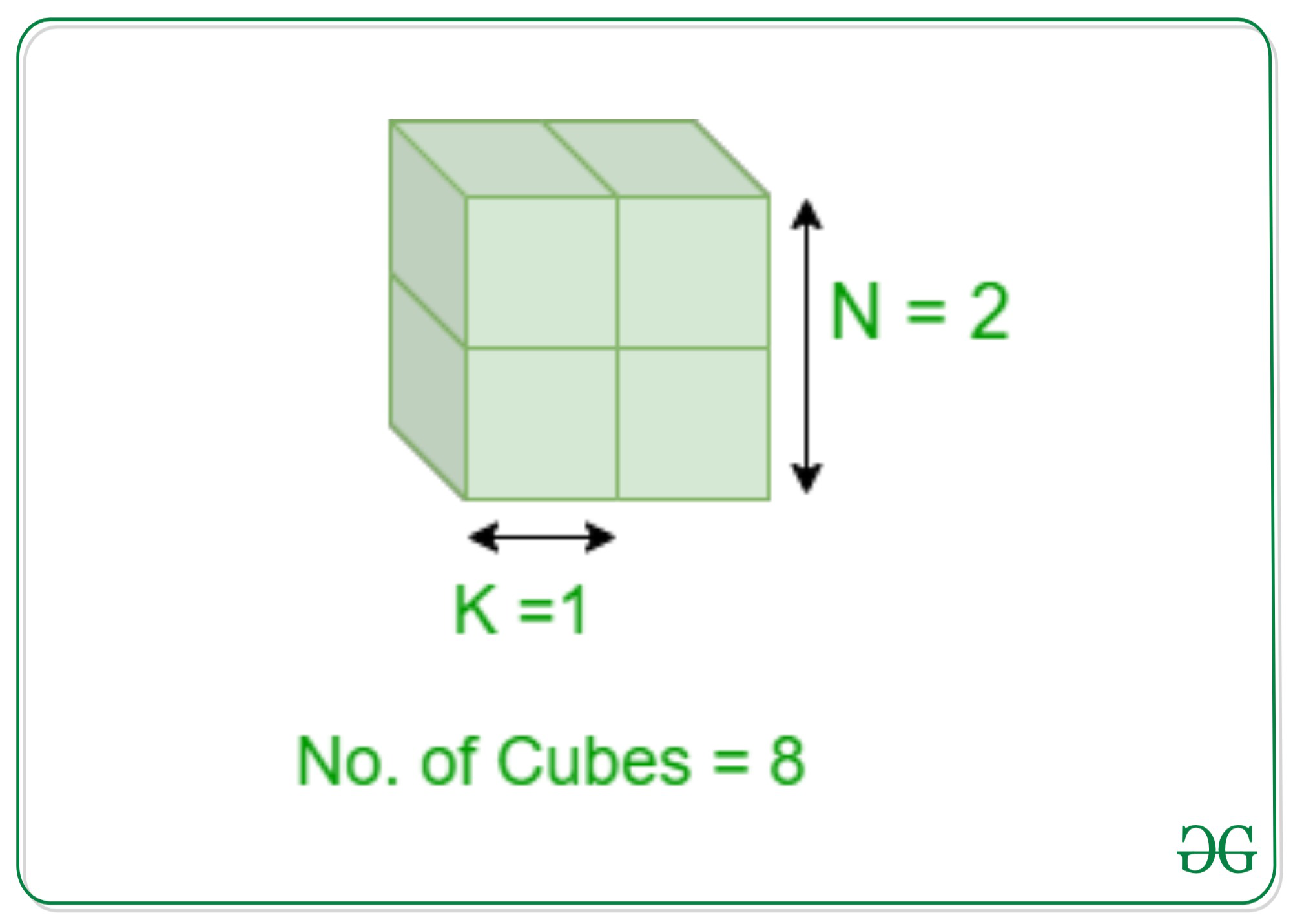# Count cubes of size K inscribed in a cube of size N

Given two integers N and K, the task is to find the number of cubes of size K that can be contained in a cube of size N.

Examples:

Input: N = 2, K = 1
Output: 8
Explanation:
There are 8 cubes of size 1 that can be drawn inside the bigger cube of size 2.Input: N = 5, K = 2
Output: 64
Explanation:
There are 64 cubes of size 2 can be drawn inside the bigger cube of size 5.

Approach: The key observation to solve the problem is that the number of cubes inside the cube of size N is (N2 * (N+1)2)/4. Therefore, the cubes of size K inside the cube of size N is:Below is the implementation of the above approach:

## C++

 `// C++ implementation of the` `// above approach`   `#include ` `using` `namespace` `std;`   `// Function to find the number` `// of the cubes of the size K` `int` `No_of_cubes(``int` `N, ``int` `K)` `{` `    ``int` `No = 0;`   `    ``// Stores the number of cubes` `    ``No = (N - K + 1);`   `    ``// Stores the number of cubes` `    ``// of size k` `    ``No = ``pow``(No, 3);` `    ``return` `No;` `}`   `// Driver Code` `int` `main()` `{` `    ``// Size of the bigger cube` `    ``int` `N = 5;`   `    ``// Size of the smaller cube` `    ``int` `K = 2;`   `    ``cout << No_of_cubes(N, K);` `    ``return` `0;` `}`

## Java

 `// Java implementation of the` `// above approach` `class` `GFG{`   `// Function to find the number` `// of the cubes of the size K` `static` `int` `No_of_cubes(``int` `N, ` `                       ``int` `K)` `{` `  ``int` `No = ``0``;`   `  ``// Stores the number of cubes` `  ``No = (N - K + ``1``);`   `  ``// Stores the number of cubes` `  ``// of size k` `  ``No = (``int``) Math.pow(No, ``3``);` `  ``return` `No;` `}`   `// Driver Code` `public` `static` `void` `main(String[] args)` `{` `  ``// Size of the bigger cube` `  ``int` `N = ``5``;`   `  ``// Size of the smaller cube` `  ``int` `K = ``2``;`   `  ``System.out.print(No_of_cubes(N, K));` `}` `}`   `// This code is contributed by Princi Singh`

## Python3

 `# Python3 implementation of the` `# above approach` ` `  `# Function to find the number` `# of the cubes of the size K` `def` `No_of_cubes(N, K):` `    `  `    ``No ``=` `0` ` `  `    ``# Stores the number of cubes` `    ``No ``=` `(N ``-` `K ``+` `1``)` ` `  `    ``# Stores the number of cubes` `    ``# of size k` `    ``No ``=` `pow``(No, ``3``)` `    ``return` `No`   `# Driver Code`   `# Size of the bigger cube` `N ``=` `5` ` `  `# Size of the smaller cube` `K ``=` `2` ` `  `print``(No_of_cubes(N, K))`   `# This code is contributed by sanjoy_62`

## C#

 `// C# implementation of the` `// above approach` `using` `System;` ` `  `class` `GFG{` `     `  `// Function to find the number` `// of the cubes of the size K` `static` `int` `No_of_cubes(``int` `N, ``int` `K)` `{` `    ``int` `No = 0;` `    `  `    ``// Stores the number of cubes` `    ``No = (N - K + 1);` `    `  `    ``// Stores the number of cubes` `    ``// of size k` `    ``No = (``int``)Math.Pow(No, 3);` `    ``return` `No;` `}` ` `  `// Driver Code` `public` `static` `void` `Main()` `{` `    `  `    ``// Size of the bigger cube` `    ``int` `N = 5;` `    `  `    ``// Size of the smaller cube` `    ``int` `K = 2;` `    `  `    ``Console.Write(No_of_cubes(N, K));` `}` `}`   `// This code is contributed by sanjoy_62`

Output:

```64

```

Time Complexity: O(1)
Auxiliary Space: O(1)

Attention reader! Don’t stop learning now. Get hold of all the important DSA concepts with the DSA Self Paced Course at a student-friendly price and become industry ready.

My Personal Notes arrow_drop_upIf you like GeeksforGeeks and would like to contribute, you can also write an article using contribute.geeksforgeeks.org or mail your article to contribute@geeksforgeeks.org. See your article appearing on the GeeksforGeeks main page and help other Geeks.

Please Improve this article if you find anything incorrect by clicking on the "Improve Article" button below.

Improved By : princi singh, sanjoy_62